Filter By

## All Questions

#### Write ‘True’ or ‘False’ and justify your answer in each of the following AB is a diameter of a circle and AC is its chord such that BAC = 30°. If the tangent at C intersects AB extended at D, then BC = BD.

Solution: First of all we solve the question according to give conditions. If we able to prove it then it will be true otherwise it will be false.
Given :BAC =
Diagram : Construct figure according to given conditions then join BC and OC.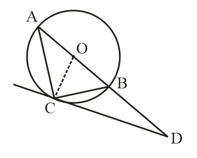To Prove : BC = BD
Proof :BAC =    (Given)

[ angle between chord and tangent id equal to the angle made by chord in alternate segment]

[ Radius and tangent’s angle is always ]
In OAC
OA = OC     (both are radius of circle)

[opposite angles of an isosceles triangle is equal]

In

[ sum of interior angle of a trianglE ]

In BCD we conclude that
and
[sides which is opposite to equal angles is always equal]
Hence Proved.
Hence the given statement is true.

#### Write ‘True’ or ‘False’ and justify your answer in each of the following  If a number of circles pass through the end points P and Q of a line segment PQ, then their centres lie on the perpendicular bisector of PQ.

Solution
According to question.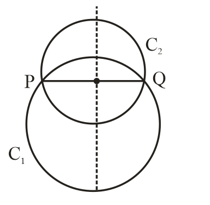Here C1, C2 circles pass through the point P and Q.
We know that the perpendicular bisector of chord of a circle is always passes through the center of the circle. Hence the perpendicular bisector of line PQ passes through the center of circles of C1, C2.
Hence the given statement is True.

## Crack CUET with india's "Best Teachers"

• HD Video Lectures
• Unlimited Mock Tests
• Faculty Support#### Write ‘True’ or ‘False’ and justify your answer in each of the following If a number of circles touch a given line segment PQ at a point A, then their centres lie on the perpendicular bisector of PQ.

Solution
According to question.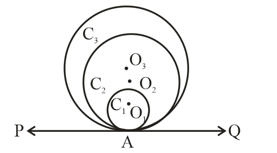Here C1, C2, C3 are the circle with center O1, O2, O3 respectively.
C1, C2, C3 touches line PQ at point A. Here PQ is the tangent at each circle.
If we join O1, O2, O3 to point A then the line is perpendicular to line PQ because if we draw a line from the center of circle at any point then the tangent at that point is perpendicular to the radius.
But here line joining the centers is not bisecting the line PQ because it depend on the length of PQ.
Hence the given statement is False.

#### Write ‘True’ or ‘False’ and justify your answer in each of the following :The tangent to the circumcircle of an isosceles triangle ABC at A, in which AB = AC, is parallel to BC.

Solution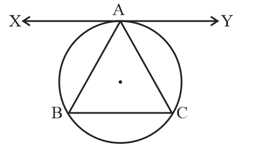Given ABC is a isosceles triangle and AB = AC.

To Prove :
Proof : AB = AC     (Given)

[  angle between chord of a circle and tangent is equal to angle made by chord in alternate segment]
Also

i.e ,
Hence true

## Crack NEET with "AI Coach"

• HD Video Lectures
• Unlimited Mock Tests
• Faculty Support#### Write ‘True’ or ‘False’ and justify your answer in each of the following :If angle between two tangents drawn from a point P to a circle of radius a and centre O is , then

Solution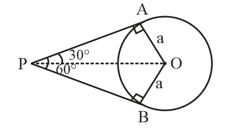Given
Draw line OP from point O to P which bisect P. Which bisect
i.e,
In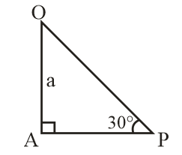OP = 2a

Hence the value of OP = 2a
Hence the given statement is False.

#### Write ‘True’ or ‘False’ and justify your answer in each of the following :If angle between two tangents drawn from a point P to a circle of radius a and centre O is , then

Solution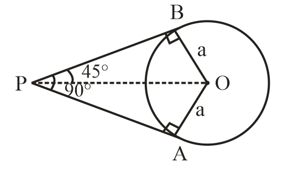Given
Draw line OP from point O to P which bisect .
i.e ,
In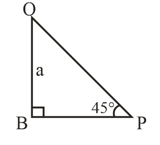Hence the given statement is True.

## Crack JEE Main with "AI Coach"

• HD Video Lectures
• Unlimited Mock Tests
• Faculty Support#### Write ‘True’ or ‘False’ and justify your answer in each of the following :The angle between two tangents to a circle may be .

Solution
It is possible that the angles of two line may be  in only two conditions.
1. When lines are parallel
2.When both the lines are coincide.
Hence the given statement is True
This may be possible only when tangents are parallel or when both the tangents are coincide.

#### Write ‘True’ or ‘False’ and justify your answer in each of the following :The length of tangent from an external point P on a circle with centre O is always less than OP.

Solution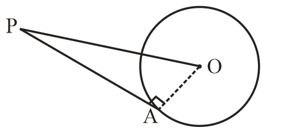Here O is the center of given circle and PA is tangent which is drawn from an external point P. OA is radius of circle.We know that tangent and radius is always perpendicular to each other.

is a right angle triangle Use Pythagoras theorem in
…..(i)
From equation (i) we can say that PA is always less than PO.
In other words we can say that the length of hypotenuse is always greater than the length of perpendicular in a right angle triangle.
i.e.,      OP  PA
Hence the given statement is True.

## Crack CUET with india's "Best Teachers"

• HD Video Lectures
• Unlimited Mock Tests
• Faculty Support#### Write ‘True’ or ‘False’ and justify your answer in each of the following :The length of tangent from an external point on a circle is always greater than the radius of the circle.

Solution
Case-I – When external point P is very close to circle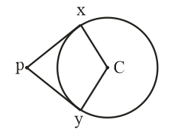Here C is the center of circle. Let this radius is 5 and distance of Px and Py is 3.Or we can say that Case-I contradict the given statement.
Case-II – When external point P is far the circle.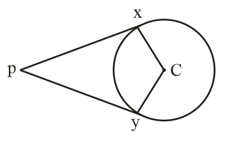Here radius of the given circle is 5 cm and let the length of Px and Py is 10 cm.
Hence according to Case-II and given statement is True.
Hence from the above two cases we conclude that the tangent’s length is depend on the distance of external point from the circle.
Therefore given statement is False because length of tangent from an external point of a circle may or may not be greater than the radius of the circle.
Therefore the given statement is False.

#### Write ‘True’ or ‘False’ and justify your answer in each of the following : If a chord AB subtends an angle of  at the centre of a circle, then angle between the tangents at A and B is also .

Solution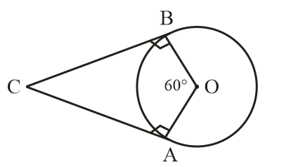Here CA and CB are the two tangents which is drawn on chord AB and also we know that tangent and radius are perpendicular to each other.
i.e.,

[  Sum of interior angles of a quadrilateral is ]

Here we conclude that angle between the tangents at A and B is .

Therefore the given statement is False.